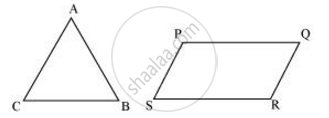# Give Two Different Examples of Pair Of Non-similar Figures - Mathematics

Give two different examples of pair of Non-similar figures

#### Solution

Trapezium and squareTriangle and parallelogramConcept: Similar Figures
Is there an error in this question or solution?
Chapter 6: Triangles - Exercise 6.1 [Page 122]

#### APPEARS IN

NCERT Class 10 Maths
Chapter 6 Triangles
Exercise 6.1 | Q 2.1 | Page 122

Share